# 1.本周学习总结

## 1.1思维导图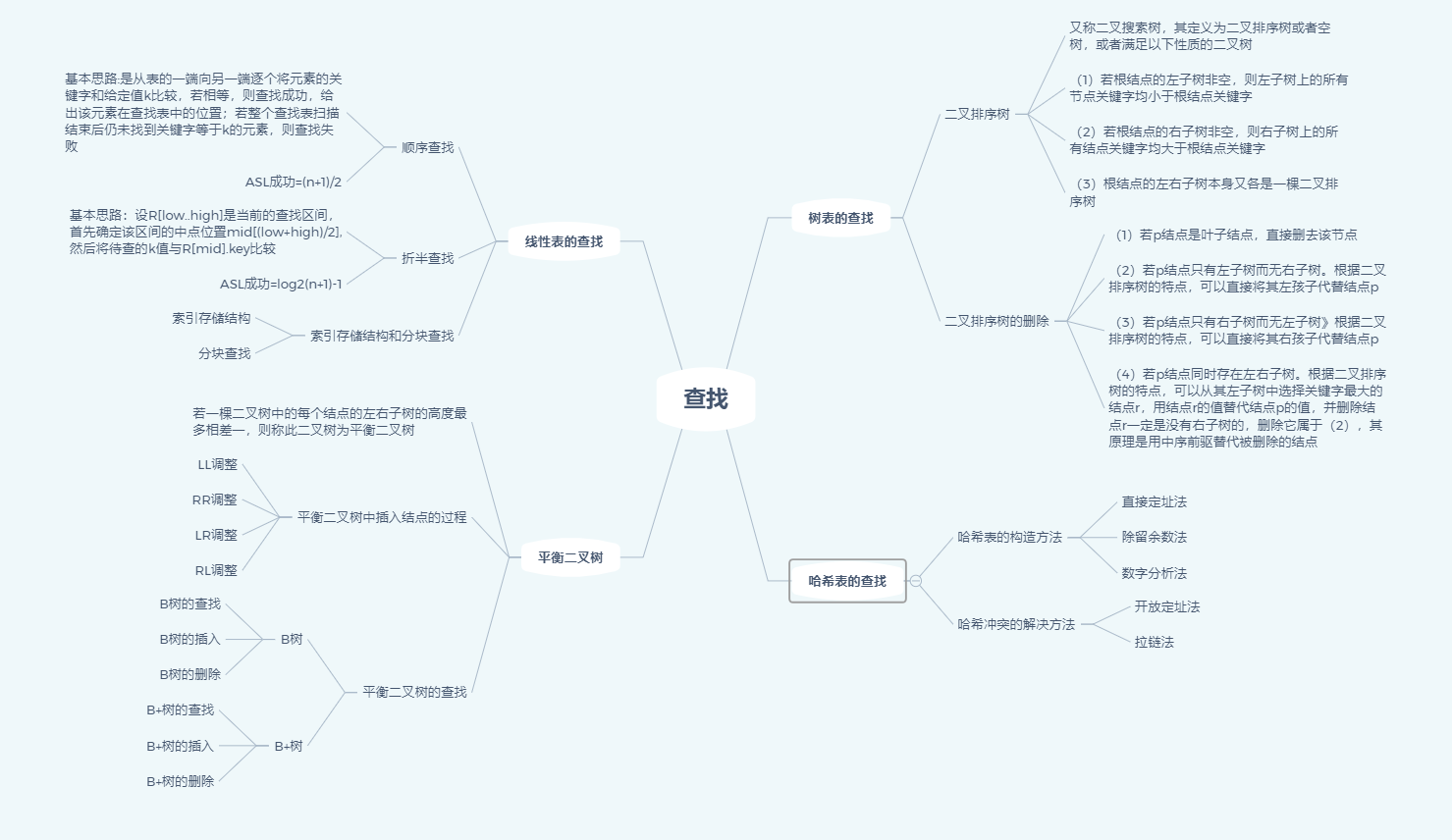## 1.2谈谈你对查找运算的认识及学习体会。，

1.查找，可以分为线性表的查找、树表的查找和哈希表的查找，而线性表则是最简单的查找表，又可分为顺序查找、折半查找和索引存储和分块查找，折半查找在上学期就已经学过了，而且书上的代码看起来没有那么晦涩难懂，所以线性表的查找学起来相较于以前就有轻松许多，而树表的二叉排序树中打许多操作则需要用到递归，其中又引入了ASL来计算平均查找长度,我感觉这一章中最难的点就是平衡二叉树的插入与删除了，LL与RR调整比LR与RL好操作一些，两种调整方式原理都相似，都是把中间数上调。在这一章中还有B-树的删除与插入也费脑子，需要关键字移来移去，对于哈希表的查找的难度在三种查找中属于中间层次，哈希表的构造方式与哈希冲突的解决方法看一下课本就可以了。
2.在课堂中，老师还介绍了map容器，如果数据和数据之间属于一对一的关系就可以使用map容器了，而且和queue、stack容器一样使用起来十分方便，不用写过多的函数来写其他功能。

# 2.PTA实验作业

## 2.1.题目1：6-1 二叉搜索树的操作集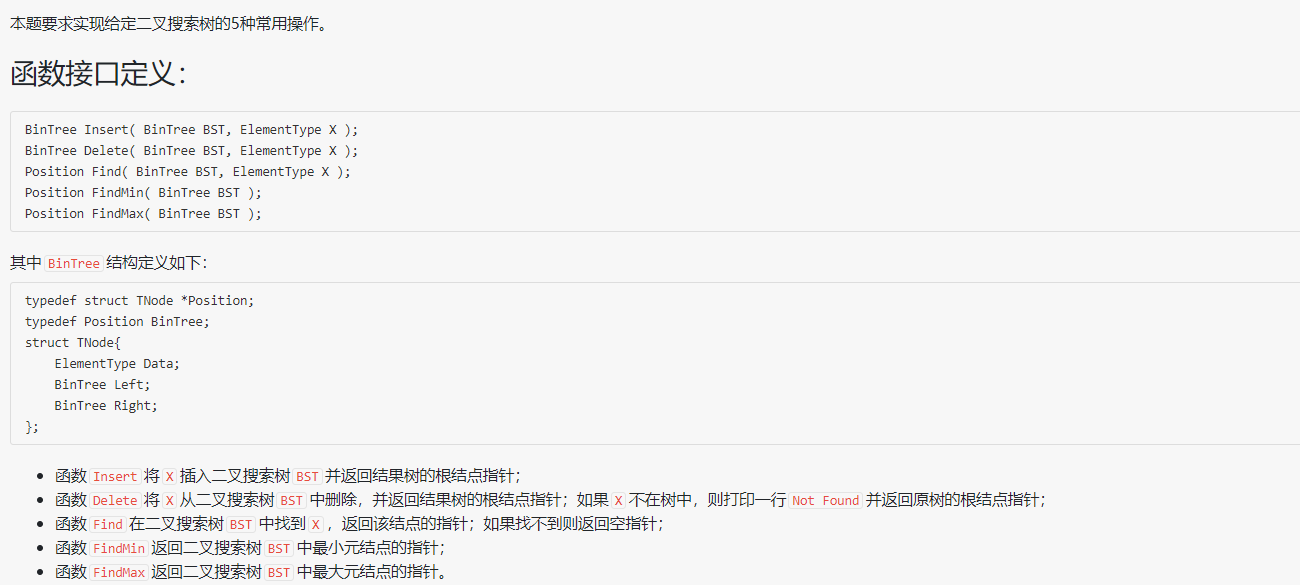### 2.1.1设计思路

Position FindMin(BinTree BST)

if BST 不为空 then
if  BST的左孩子不空 then
递归调用函数 FindMin(BST->Left);

else
返回BST;
end if

Position FindMax(BinTree BST)

if  BST 不为空  then
if BST右孩子不为空 then
while BST右孩子不为空
BST = BST->Right;
end while
end if
end if
返回 BST;

BinTree Insert(BinTree BST, ElementType X)

if  BST为空 then
定义BinTre类型结点p;
申请动态空间;
X存入p的数据域;
p的左右孩子初始化为空;
BST = p;
else if X < BST->Data then
BST->Left = Insert(BST->Left, X);
else if X < BST->Data then
BST->Right = Insert(BST->Right, X); //利用递归来进行数据的插入
end if
返回 BST;

BinTree Delete(BinTree BST, ElementType X)

if BST 为空 then
else
if  X < BST->Data then
BST->Left = Delete(BST->Left, X);
else if  X > BST->Data then
BST->Right = Delete(BST->Right, X);
else if X = BST->Data
if  BST的左右孩子都不为空 then
新建BinTree类型结点p;
p = FindMin(BST->Right);
BST->Data = p->Data;
BST->Right = Delete(BST->Right, BST->Data);

else
if BST的左孩子为空 then
BST = BST->Right;
else if BST右孩子为空 then
BST = BST->Left;
end if
end if
end if
返回 BST;

Position Find(BinTree BST, ElementType X)

if  BST为空 then
返回空值;
if BST->Data == X then
返回BST结点;
else if X < BST->Data then
利用递归找下一个左孩子结点;
else if (X > BST->Data)
利用递归找下一个左孩子结点;
返回BST;


### 2.1.2代码截图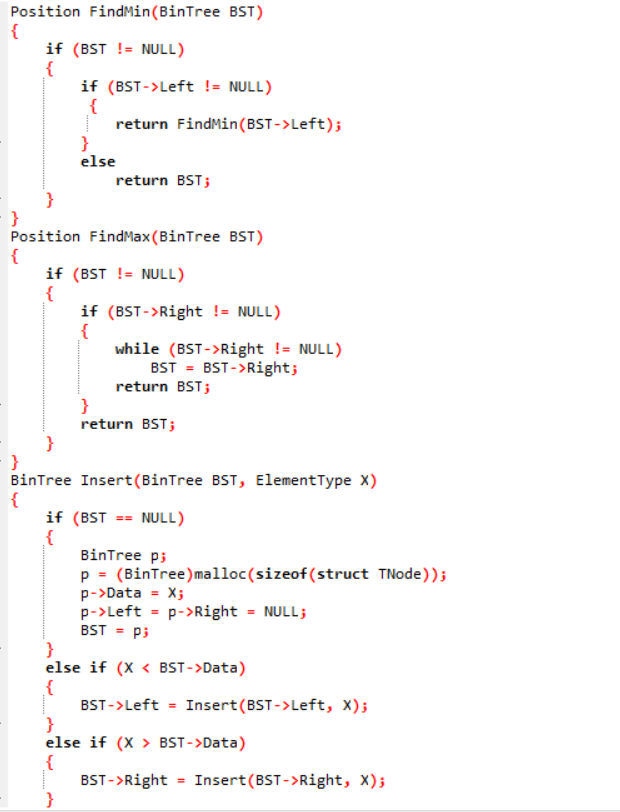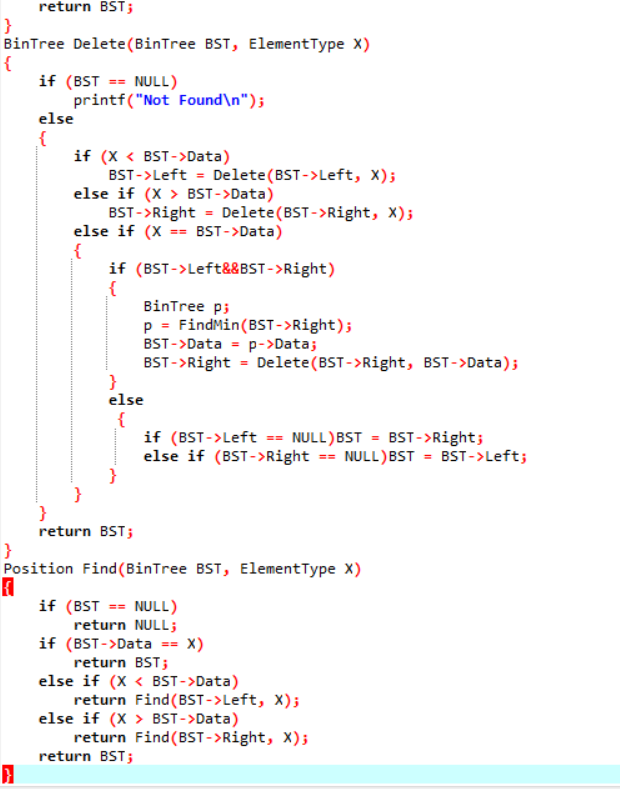### 2.1.3本题PTA提交列表说明。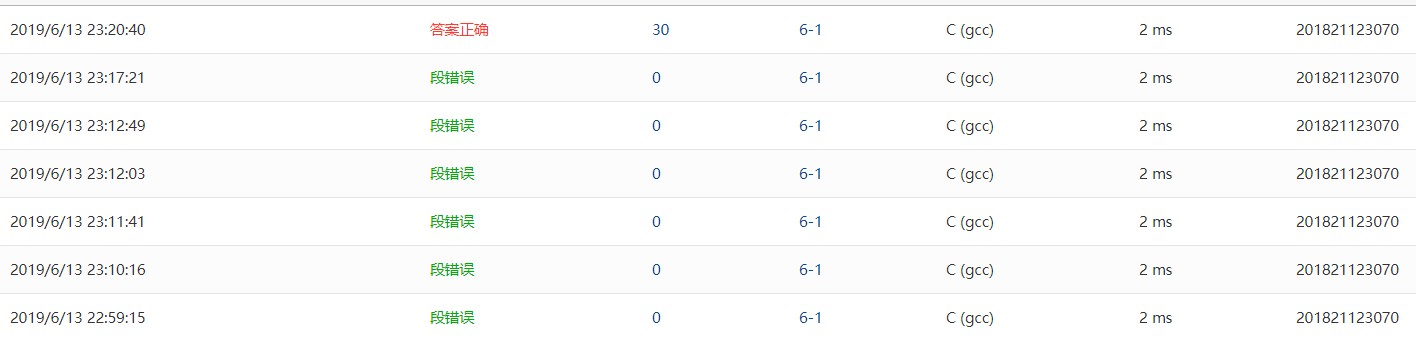1.在刚开始写的时候，是边看书边写的，然后放在pta上老是出现段错误，也是很无奈，发现在二叉排序树的删除少了一个结点来存放未被删除的结点，程序发生奔溃；
2.后来在网上找了一段二叉排序树的删除代码，理解了一遍，自己写一遍，调试了很久觉得思路就是没毛病，后面看到有一个指针指错了。

## 2.2.题目2

6-3 二叉搜索树中的最近公共祖先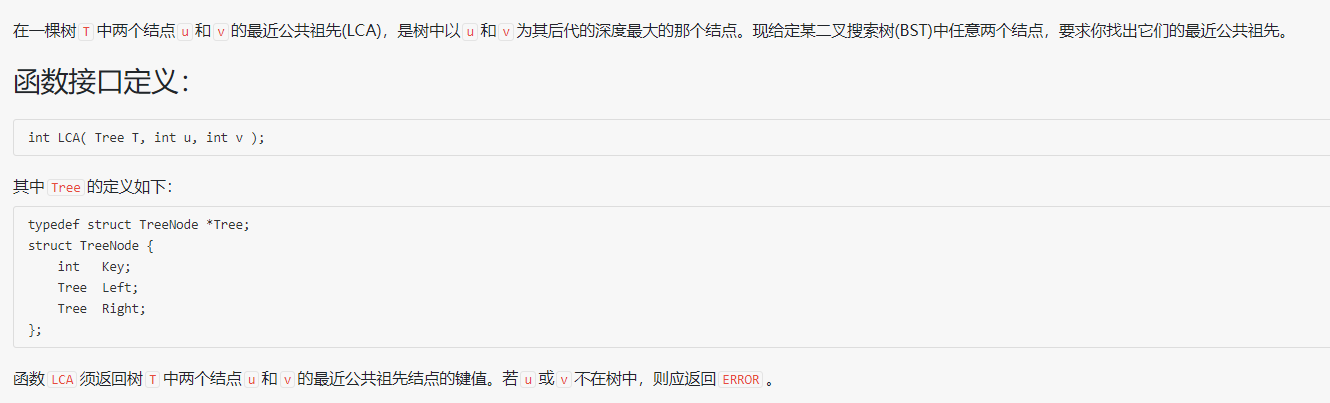### 2.2.1设计思路

int find(Tree T, int x)

if  T为空 then
return 0
if  x 等于 关键字 then
return 1
else if  x 大于 关键字
利用递归找下一个左孩子结点;
else
利用递归找下一个右孩子结点;

int LCA(Tree T, int u, int v)

if  T 为空 then
返回ERROR
if  find(T, u)=0或者find(T, v)=0  then //如果找不到这两个结点
返回ERROR
if T->Key>=u&&T->Key<v||T->Key<=u&&T->Key>=v then
返回 T->Key
if u > T->Key then                 //使用递归找下一个结点
LCA(T->Right, u, v);
else if then u < T->Key then
LCA(T->Left, u, v);


### 2.2.2代码截图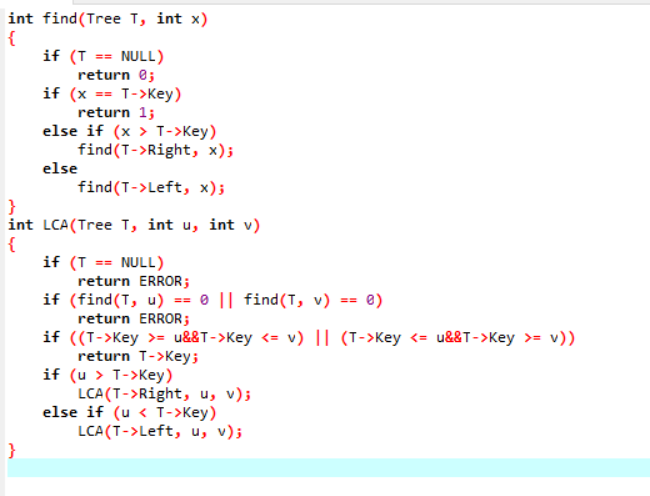### 2.2.3本题PTA提交列表说明。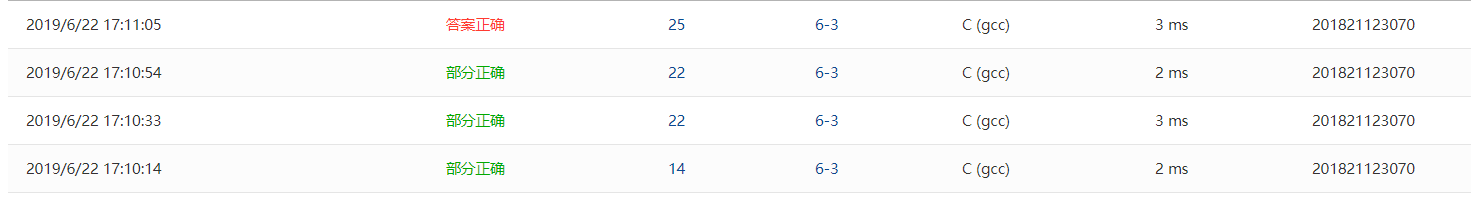1.不知道祖宗节点的条件是什么，只写出了空树样例，后来考到课本上二叉排序树的特点：若它的左子树不空，则左子树上所有结点的值均小于它的根结点的值；

2.忘记了两个数据都不存在的情况。

## 2.3.题目3：

7-1 QQ帐户的申请与登陆

### 2.3.1设计思路

定义变量a来存储命令符,定义变量b来存储账号,定义变量c来储存密码;

for i=0 to n do

输入命令符a,账号b,密码c;

if 命令符a==L then                       //登入状态
if myMap.find(b) == myMap.end() then //如果账号在mymap里找不到
输出:ERROR: Not Exist;
if myMap[b] != c then                //如果账号与密码不匹配
输出:ERROR: Wrong PW;
else
end if

if 命令符a ==N then                      //注册状态
if myMap.find(b)!= myMap.end() then  //如果账号在mymap里找得到
输出:ERROR: Exist;
else
输出:New: OK;
myMap[b] = c;                   //将账号与密码象配对
end if

end for



### 2.3.2代码截图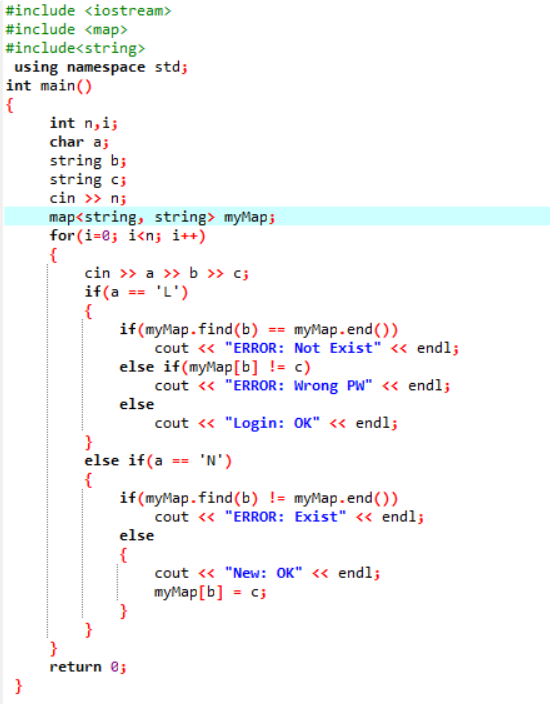### 2.3.3本题PTA提交列表说明。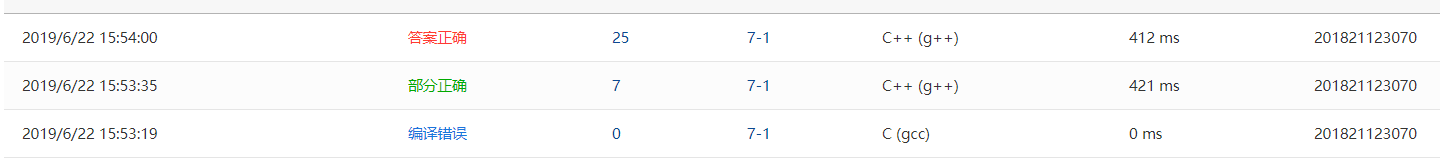1.刚开始写用本来想用两个个数组将数据储存起来，与输入的数据相比较，想去年课设一样，但是太过复杂且没有文件将数据导出导入，遂放弃；
2.老师上课讲过用map容器来写，然后在网上找了关于map容器的函数，但是由于不熟练，将myMap[b] = c写成了了c=myMap[b]导致myMap中永远没有数据，测试点过的永远是ERROR: Not Exist这个测试点；

# 3、阅读代码

## 3.1 题目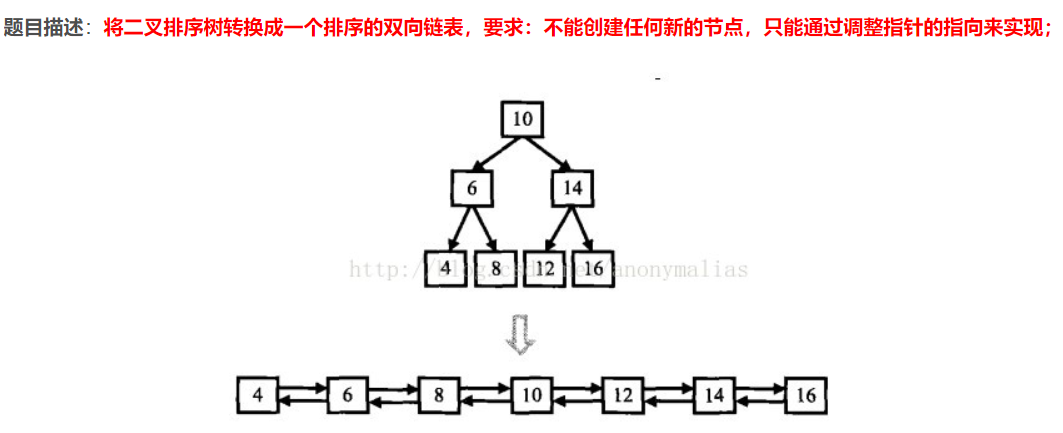## 3.3 代码截图

#include <iostream>
#include <iterator>
#include <algorithm>
using namespace std;

struct BSTreeNode
{
int  nValue;
BSTreeNode*  left;
BSTreeNode*  right;
BSTreeNode(int n)
{
nValue = n;
left = NULL;
right = NULL;
}
};

//前一个节点指针
BSTreeNode* gPreNode = NULL;

//头节点

{
if (pNode == NULL)
{
pNode = new BSTreeNode(nNode);
pNode->left = NULL;
pNode->right = NULL;
}
else if (pNode->nValue > nNode)
{
}
else if(pNode->nValue < nNode)
{
}
else
{
cout << "Same Node" << endl;
}
}

void TransToListNode(BSTreeNode* pNode)
{
if (gPreNode == NULL)
{
gPreNode = pNode;
return;
}

gPreNode->right = pNode;
pNode->left = gPreNode;

gPreNode = pNode;
}

{
{
cout << pHead->nValue << " ";
}

cout << endl;
}

void MidOrderPrint(BSTreeNode* pRoot)
{
if (pRoot == NULL)
{
return;
}

MidOrderPrint(pRoot->left);
//cout << pRoot->nValue << " ";
TransToListNode(pRoot);

MidOrderPrint(pRoot->right);
}

int main()
{
BSTreeNode* pRoot = new BSTreeNode(100);

 这一题将指针的应用发挥的淋漓尽致，这一题中使用了左右孩子结点指针，前后继结点指针，各种指针指来指去，如果没有认真去看，可能光节点名就能把人搞晕，不过建立二叉排序树函数还是和课本相类似的，都是运用了递归，而核心部分的转化双向链表的前继结点和后继结点则是用二叉树的左右结点来表示，这里定义了一个prenode来来储存前继结点，然后prenode指针后移来建立双向链表，right和left结点既可以用来表示二叉树的左右孩子又可以表示双向链表的前后结点，感觉作者肯定花了不少心思，这一题整体上就是将二叉排序树和链表知识一起运用，需要对链表和二叉排序树的结构十分了解。